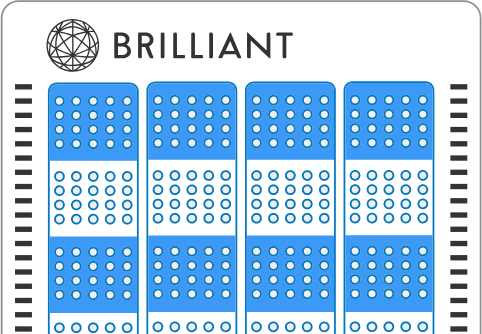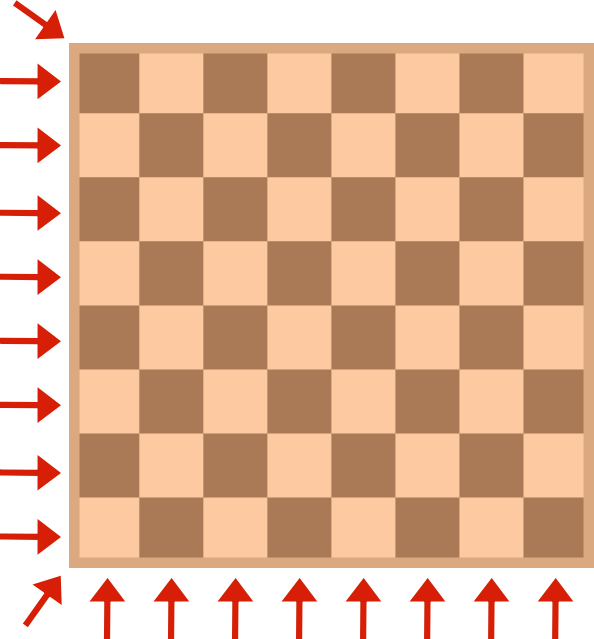### Contest Math II

In addition to learning about various methods and formulas, this course will also expose you to meta-strategies for problem-solving. Some of these apply generally; others are specifically for math contests at the level of the AMC.# Key Strategies

Often, it's useful to think about an extreme case of a problem. Then, you can check if that extreme case is possible, and if not, how close you can get.

What is the maximum number of coins that can be placed on squares in an $8\times 8$ chessboard such that each row, each column, and each long diagonal contains at most 4 coins? (Note: Only 1 coin is allowed per square.)# Key Strategies

When solving questions about a general variable, it can be useful to test small cases and then make a conjecture about the overall problem.

Suppose $p$ is a prime number such that $p^2+11$ has exactly six different positive divisors $($including $1$ and the number itself$).$ What is the sum of all possible values of $p?$
(Note: There may be more than one possible value.)

# Key Strategies

When a problem involves some sort of symmetry, make use of it!If $6$ fair coins are flipped, what is the probability that there are more heads than tails?

Hint: What is the probability that the same number of heads and tails are flipped, and what are the other possibilities?

# Key Strategies

In math contests, eliminating impossible or unlikely choices makes guessing much more beneficial.

You encounter the question below, but your paper has been smudged. Nonetheless, you can still answer the question correctly!For all positive integers $n,$ the expression above is always divisible by all of the following positive integers except one. Which one?

# Key Strategies

Casework is a wide-reaching strategy of breaking a complex problem down into multiple cases - each of which is relatively simple - and then combining them to obtain the overall result.

Find the sum of all solutions to the equation

$\large (x^2+5x+5)^{x^2-10x+21}=1 .$

Hint: Don't forget about the case where the base is $-1$ and the exponent is even!

# Key Strategies

These introductory quizzes have given you just a glimpse of what's to come in Contest Math II.

Whether you're looking for a comprehensive guide $($with over $50$ quizzes in a wide range of topics$)$ or just looking to brush up on some specific techniques, there's something here for you.

The last two quizzes of this introduction involve deep problem solving of the sort you'll find on the AMC and other contest math situations.

Dive in, and good luck!

×